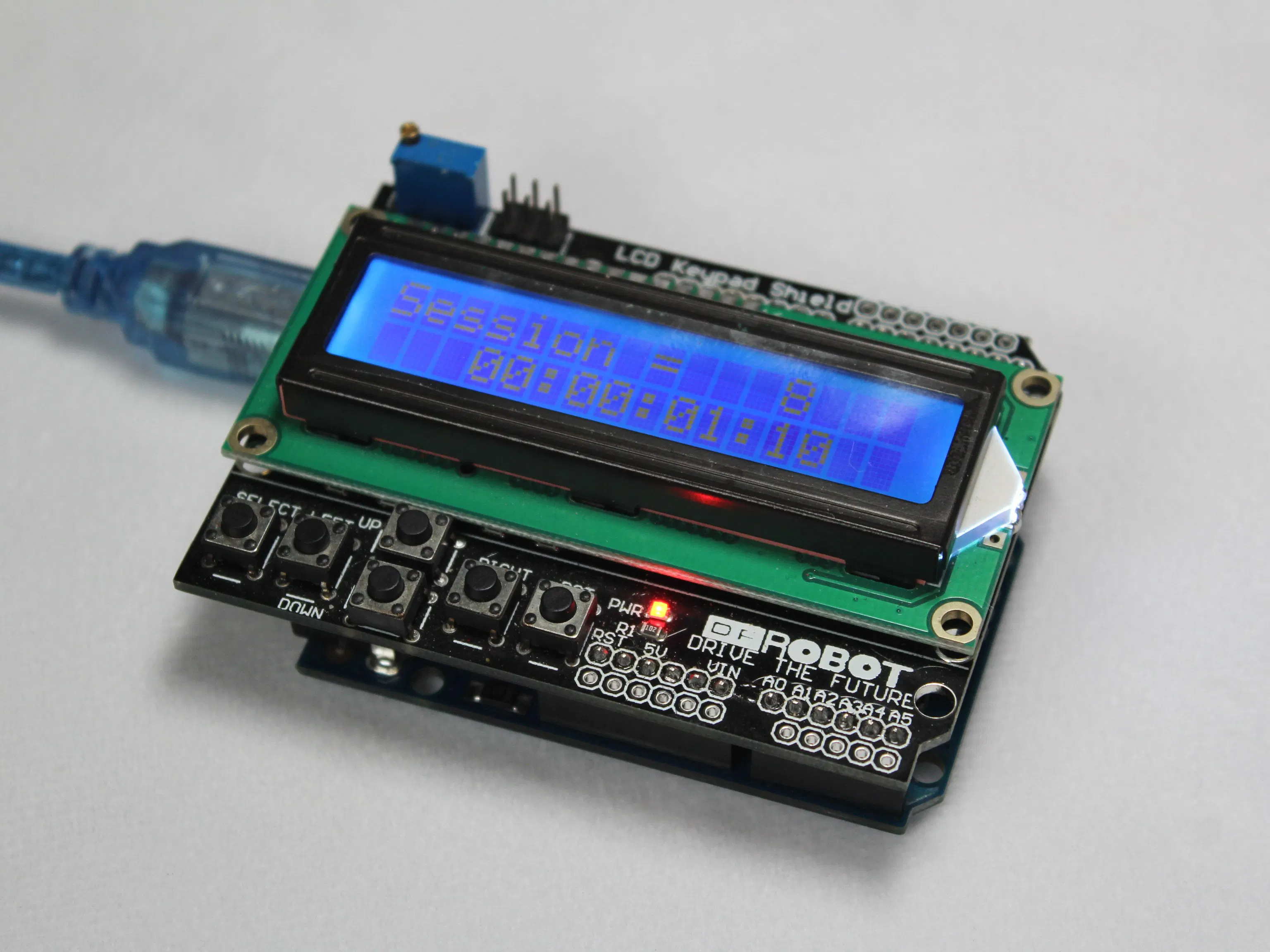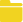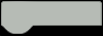# Arduino UNO Power Time Logger

Records each time a USB port is powered on/off and logs the data for up to 24 hours.Things used in this project

### Hardware components

HARDWARE LIST
1 Arduino UNO
1 DFRobot 1602 LCD Keypad Shield
1 USB-A to B Cable

Arduino IDE

### Hand tools and fabrication machines

3D Printer (generic)

StoryPower Time Logger 1.0.0 for Arduino UNO and LCD Keypad Shield.

Created on October 7, 2022

Power Time Logger records each time a USB port is powered on/off.

Total recording time is 365 days.

Press the SELECT button to switch between total on/off and total time.

Press the LEFT button to reset the program.

Use this project to log usage times for any device that has an on/off USB port (wi-fi router, 3D printer, computer, etc.).

This software is free and open-source; anyone can redistribute it and/or modify it.

Custom parts and enclosures

### Arduino LCD Shield Enclosure Caseparts_RIKVOyInAy.zip 497KB Download(0)

Code

### Power Time Logger (365 days)

C/C++

Power Time Logger 1.0.0 for Arduino UNO and LCD Keypad Shield.

CODE
``````/*
Power Time Logger 1.0.0 for Arduino UNO and LCD Keypad Shield.
Created on October 23, 2022
www.alexaldridge.co

Power Time Logger records each time a USB port is powered on/off.
Total recording time is 365 days.

Press the SELECT button to switch between total on/off and total time.
Press the LEFT button to reset the program.

Use this project to log usage times for any device that has an on/off USB port (wi-fi router, 3D printer, computer, etc.).

This software is free and open-source; anyone can redistribute it and/or modify it.
*/

#include <LiquidCrystal.h>
#include <EEPROM.h>

const int pin_RS = 8;
const int pin_EN = 9;
const int pin_d4 = 4;
const int pin_d5 = 5;
const int pin_d6 = 6;
const int pin_d7 = 7;
const int pin_BL = 10;
LiquidCrystal lcd( pin_RS,  pin_EN,  pin_d4,  pin_d5,  pin_d6,  pin_d7);
extern volatile unsigned long timer0_millis;

int count_turn_on=0;
unsigned long storage;
unsigned long seconds ;
unsigned long minutes ;
unsigned long hours ;
unsigned long days;
unsigned long runtime;
unsigned long runtime_total;
unsigned long future;
unsigned long total_acc_time;

void setup() {
lcd.begin(16, 2);

count_turn_on=count_turn_on+1;
EEPROM.write(0, count_turn_on);

EEPROM_writelong(0x55, future);
EEPROM_writelong(0x02,0);
}

void loop() {
runtime=millis();
runtime_total=runtime;

lcd.setCursor(0, 0);
lcd.print("Session: ");
lcd.setCursor(10, 0);

seconds = runtime / 1000;
minutes = seconds / 60;
hours = minutes / 60;
days=hours / 24;
runtime %= 1000;
seconds %= 60;
minutes %= 60;
hours %= 24;
days %=1;
lcd.setCursor(0,1);
lcd.print("T ");
if(days<100)
{
lcd.setCursor(3, 1);
lcd.print("0");
lcd.setCursor(4,1);
lcd.print(days);
}
if(days<10)
{
lcd.setCursor(3, 1);
lcd.print("00");
lcd.setCursor(5,1);
lcd.print(days);
}
if(days>10)
{
lcd.setCursor(3, 1);
lcd.print("0");
lcd.setCursor(4,1);
lcd.print(days);
}
if(days>100)
{
lcd.setCursor(3,1);
lcd.print(days);
}
lcd.setCursor(6, 1);
lcd.print(":");
if(hours<10)
{
lcd.setCursor(7, 1);
lcd.print("0");
lcd.setCursor(8,1);
lcd.print(hours);
}
else if(hours>=10)
{
lcd.setCursor(7,1);
lcd.print(hours);
}
lcd.setCursor(9, 1);
lcd.print(":");
if(minutes<10)
{lcd.setCursor(10, 1);
lcd.print("0");
lcd.setCursor(11,1);
lcd.print(minutes);
}else if (minutes>=10)
{lcd.setCursor(10,1);
lcd.print(minutes);
}
lcd.setCursor(12, 1);
lcd.print(":");
if (seconds<10)
{
lcd.setCursor(13, 1);
lcd.print("0");
lcd.setCursor(14, 1);
lcd.print(seconds);
}
else if (seconds>=10)
{
lcd.setCursor(13, 1);
lcd.print(seconds);
}

if (runtime>=8208000 )
{
noInterrupts ();
timer0_millis = 0;
interrupts ();
}

EEPROM_writelong(1x02,runtime_total);

{
lcd.clear();
total_acc_time=future+runtime_total;

seconds = total_acc_time / 1000;
minutes = seconds / 60;
hours = minutes / 60;
days=hours / 24;
total_acc_time %= 1000;
seconds %= 60;
minutes %= 60;
hours %= 24;
days %=1;
lcd.setCursor(3,0);
lcd.print("Total time");
lcd.setCursor(0,1);
lcd.print("");

if(days<100)
{
lcd.setCursor(3, 1);
lcd.print("0");
lcd.setCursor(4,1);
lcd.print(days);
}
if(days<10)
{
lcd.setCursor(3, 1);
lcd.print("00");
lcd.setCursor(5,1);
lcd.print(days);
}
if(days>10)
{
lcd.setCursor(3, 1);
lcd.print("0");
lcd.setCursor(4,1);
lcd.print(days);
}
if(days>100)
{
lcd.setCursor(3,1);
lcd.print(days);
}
lcd.setCursor(6, 1);
lcd.print(":");

if(hours<10)
{
lcd.setCursor(7, 1);
lcd.print("0");
lcd.setCursor(8,1);
lcd.print(hours);
}
else if(hours>=10)
{
lcd.setCursor(8,1);
lcd.print(hours);
}
lcd.setCursor(9, 1);
lcd.print(":");
if(minutes<10)
{lcd.setCursor(10, 1);
lcd.print("0");
lcd.setCursor(11,1);
lcd.print(minutes);
}else if (minutes>=10)
{lcd.setCursor(10,1);
lcd.print(minutes);
}
lcd.setCursor(12, 1);
lcd.print(":");
if (seconds<10)
{
lcd.setCursor(13, 1);
lcd.print("0");
lcd.setCursor(14, 1);
lcd.print(seconds);
}
else if (seconds>=10)
{
lcd.setCursor(13, 1);
lcd.print(seconds);
}
delay(1000);
lcd.clear();
}
//
{
lcd.clear();
EEPROM_writelong(0x02,0);
EEPROM_writelong(0x55,0);
total_acc_time=0;
future=0;
EEPROM.write(0, 0);
noInterrupts ();
timer0_millis = 0;
interrupts ();
lcd.setCursor(0,0);
lcd.print("Reset");
delay(1000);
lcd.clear();
}
}
{

dword = dword << 16;

return dword;
}

{
}

void EEPROM_writelong(int address, unsigned long value)
{
value = value >> 16;
}

{
return word;
}``````

The article was first published in hackster, October 7, 2022

cr: https://www.hackster.io/alexaldridge/power-time-logger-for-arduino-uno-fe7fab

author: alexaldridge